## 1 Introduction

Model-based design has been widely used, particularly in the design of safety-critical real-time embedded systems. It has achieved industrial successes through languages such as SCADE , AADL  and UML MARTE . For example, UML MARTE provides syntactic annotations to implement, when the context allows, classical real-time scheduling algorithms such as EDF (Earliest Deadline First). It also provides a domain-specific language–Clock Constraint Specification Language (CCSL) , to express the real-time behaviors of a system under development as logical constraints on system events, but independently of any physical time and classical real-time scheduling algorithms. CCSL has been used on several industrial scenarios such as vehicle systems  and cyber-physical systems [10, 22].

Model-based design usually starts with coarse-grained logical models that are progressively refined into more concrete ones until the final code deployment. It is well-known that the earlier one can detect and fix bugs in the refinement process, the better . Therefore, it is critical to provide efficient methods and tools to check safety, liveness and schedulability on the logical models and not only on the definite deployed system. This has motivated a large body of works on verifying whether events are schedulable under a set of constraints expressed in CCSL  [11, 21, 28, 33, 35, 36, 38], though its decidability is still open. These works first transform CCSL constraints into other formal representations such as transition systems , Promela , Büchi automata , timed automata , rewriting logics , instant relations , or timed-interval logics , and then apply existing tools. However, their approaches usually suffer from the state explosion problem. Moreover, most of these works only deal with the so-called safe subset of CCSL and the other ones only provide semi-algorithms. In our earlier work , we proposed an SMT-based verification approach to CCSL and demonstrated several applications of the approach to finding schedules, verifying temporal properties, proving constraint entailment, and analyzing the validity of system traces. Based on the approach, we implemented an efficient tool for verifying LTL properties of CCSL .

In this work we are focused on the scheduling problem of CCSL, a fundamental problem to which the aforementioned verification problems of CCSL can be reduced. We first prove that the bounded scheduling problem of CCSL with fixed bounds is NP-complete. To our knowledge, this is the first result regarding the complexity of the scheduling problem with CCSL. Then, we propose a decision procedure for the bounded scheduling problem with a given bound. The decision procedure is based on the transformation of CCSL into SMT formulas . Our decision procedure is sound, complete, and efficient in practice. Based on this decision procedure, we turn to the general (i.e. unbounded) scheduling problem and present a binary-search based algorithm. Our algorithm is sound, i.e., if it proves either schedulable or unschedulable, then the result is conclusive. We implemented our algorithms in a prototype tool. The tool was used to analyze a real-world interlocking system in a rail transit system. Using the proposed approach, we also prove some algebraic properties of CCSL. The experimental results demonstrate the effectiveness and efficiency of the SMT-based approach.

The rest of this paper is organised as follows: Section 2 introduces CCSL. Section 3 defines the (bounded) scheduling problem of CCSL and shows that the bounded case is NP-complete. Section 4 presents an SMT-based decision procedure for the bounded scheduling problem and a sound algorithm for the general scheduling problem. Section 5 shows a case study and experimental results. Section 6 discusses related work, and Section 7 concludes the paper.

## 2 The Clock Constraint Specification Language

### 2.1 Logical Clock, History and Schedule

In CCSL, clocks are used to model occurrences of events, where a clock ticks when the corresponding event occurs. For instance, a clock may represent an event that is dispatch of a task, communications between tasks or acquisition of a shared resource by a task. Constraints over clocks are used to specify causal and temporal relations between system events. No global physical time is presumed for the clocks and their constraints. This feature allows CCSL to define a polychronous specification of a system at a logical level.

### Definition 1

(Logical clock). A (logical) clock c is an infinite sequence of ticks $$(c^i)_{i\in {\mathbb N}^+}$$ with each $$c^i$$ being tick or idle, where $${\mathbb N}^+$$ denotes the set of all the non-zero natural numbers.

The value of $$c^i$$ denotes whether an event associated with c occurs or not at step i. If $$c^i$$ is tick, then the event occurs, otherwise not. In particular, we denote by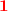a global reference logical clock that always ticks at each step.

### Definition 2

(Schedule). Given a set C of clocks, a schedule of C is a total function $$\delta :\mathbb N^{+} \rightarrow 2^{C}$$ such that $$\forall i\in \mathbb N^{+}$$, $$\delta (i)=\{c\in C \mid c^i=\textit{tick}\}$$ and $$\delta (i)\ne \emptyset$$.

Intuitively, a schedule $$\delta$$ defines a partial order between the ticks of the clocks. $$\delta (i)$$ is a subset of C such that $$c\in \delta (i)$$ iff c ticks at step i. The condition $$\delta (i)\ne \emptyset$$ expresses that step i cannot be empty. This forbids stuttering steps in schedules. As one can add or remove finite number of empty steps without effect on schedulability, we exclude them from schedules for succinctness.

A clock can memorize the number of ticks that it has made. We use history to represent the memorization.

### Definition 3

(History). Given a schedule $$\delta$$ for a set C of clocks, a history of $$\delta$$ is a function $$\chi _{\delta }:C \times \mathbb N^+ \rightarrow \mathbb N$$ such that for each $$c\in C$$ and $$i\in \mathbb N^+$$:

\begin{aligned} \chi _{\delta }(c,i)=\left\{ \begin{array}{ll} 0, &{} \mathrm{if } \,\,i=1; \\ \chi _{\delta }(c,i-1), &{} \mathrm{if }\,\, i> 1 \wedge c\not \in \delta (i-1);\\ \chi _{\delta }(c,i-1)+1, &{} \mathrm{if }\, \,i > 1 \wedge c\in \delta (i-1). \end{array} \right. \end{aligned}

$$\chi _{\delta }(c,i)$$ represents the number of the ticks that the clock c has made immediately before step i. (Note that the tick of c at step i is excluded in $$\chi _{\delta }(c,i)$$.) For simplicity, we may write $$\chi$$ for $$\chi _{\delta }$$ if it is clear from the context.

### 2.2 Syntax and Semantics of CCSL

CCSL consists of 11 kinds of constraints, 4 of them are binary relations for specifying the precedence, causality, subclocking, and exclusion relations between clocks, and the others are used to define clocks from existing ones. Clocks defined by constraints may correspond to system events or are just introduced as auxiliary clocks without corresponding to any events.

### Definition 4

(Syntax). A CCSL constraint $$\phi$$ is defined by the following form:

where $$b\ge 0$$, $$d\ge 0$$ and $$p>0$$ are natural numbers, $$c_1$$, $$c_2$$, $$c_3$$ are logical clocks and w is a (possibly infinite) word over $$\{0,1\}$$ expressed as a ($$\omega$$-)regular expression.

For simplifying presentation, we denote bythe constraint, and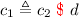the constraint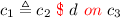such that $$c_2=c_3$$.

The semantics of CCSL constraints is defined over schedules. Given a CCSL constraint $$\phi$$ and a schedule $$\delta$$, the satisfiability relation $$\delta \models \phi$$ (i.e., $$\delta$$ satisfies constraint $$\phi$$) is defined in Table 1.

The precedence constraint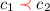(i.e.,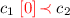) expresses that the clock $$c_1$$ precedes the clock $$c_2$$. Suppose there is an unbounded buffer with two operations fetch and store, which respectively fetch data from and store data into the buffer. Fetch is only allowed when the buffer is nonempty. If the buffer is initially empty, store operation must strictly precede fetch operation. This behavior can be expressed by the constraint: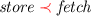. Likewise, the precedence constraint can be used to represent reentrant tasks by replacing store with start and fetch with finish.

The general precedence constraintthat can specify the differences b between the number of occurrences of two clocks before the precedence takes effect. Hence, it is able to express more complicated relations. For instance, if the buffer initially is nonempty, fetch operations can be performed prior to any store operation. Figure 1 shows such a scenario where 4 elements are initially presented in the buffer. This behavior can be represented as: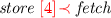.

The causality, subclock and exclusion constraints are straightforward. The causality constraint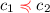specifies that the occurrence of $$c_2$$ must be caused by the occurrence of $$c_1$$, namely at any moment $$c_1$$ must have ticked at least as many times as $$c_2$$ has. The subclock constraint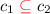expresses that $$c_1$$ occurs at some step only if $$c_2$$ occur at this step as well. The exclusion constraintspecifies that two clocks $$c_1$$ and $$c_2$$ are exclusive, i.e., they cannot occur simultaneously at the same step.

The union and intersection constraints are used to define clocks.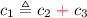defines a clock $$c_1$$ such that $$c_1$$ ticks iff $$c_2$$ or $$c_3$$ ticks. Similarly,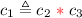defines a clock $$c_1$$ such that $$c_1$$ ticks iff both $$c_2$$ and $$c_3$$ tick. The infimum (resp. supremum) constraint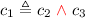(resp.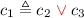) is used to define a clock $$c_1$$ that is the slowest (resp. fastest) clock that is faster (resp. slower) than both $$c_2$$ and $$c_3$$. These two constraints are useful for expressing delay requirements between two events. Remark that clocks $$c_1$$ defined by constraints may correspond to system events, otherwise are auxiliary clocks. In the former case, these constraints can be seen as constraints specifying relations between clocks $$c_1$$, $$c_2$$ and $$c_3$$.

The periodicity constraint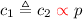defines a clock $$c_1$$ such that $$c_1$$ has to be performed once every p occurrences of clock $$c_2$$. It is worth mentioning that the periodicity constraint defined in such a way is relative because of the logical nature of CCSL clocks. That is, clock $$c_1$$ is relatively periodic with respect to clock $$c_2$$. CCSL does not assume the existence of a global reference clock, most relations are defined relative to other clocks. These notions extend the equivalent behaviors which are usually defined relative to physical time. If $$c_2$$ represents a sensor that measures physical time, then $$c_1$$ becomes physically periodic.

The filtering constraint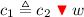is used to define a clock $$c_1$$ which can be seen as snapshots of the clock $$c_2$$ at some steps according to the ($$\omega$$-)regular expression w. For instance,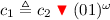expresses that $$c_1$$ simulates $$c_2$$ at every even step. It defines a logically periodic behavior of $$c_1$$ with respect to $$c_2$$.

The delayFor constraint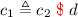(i.e.,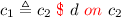) defines a new clock $$c_1$$ that is delayed by the clock $$c_2$$ with d steps. The general formdefines a new clock $$c_1$$ that is delayed by $$c_2$$ with d times of the ticks of $$c_3$$. $$c_1$$ can be seen as a sampled clock of $$c_2$$ on the basis of $$c_3$$. For instance,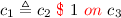, denotes that whenever $$c_2$$ ticks at least once between two successive ticks of $$c_3$$ at steps m and n, $$c_1$$ must tick at step n.

## 3 Scheduling Problem of CCSL

### 3.1 Schedulability

Given a set $$\varPhi$$ of CCSL constraints, a schedule $$\delta$$ satisfies $$\varPhi$$, denoted by $$\delta \models \varPhi$$, iff $$\delta \models \phi$$ for all constraints $$\phi \in \varPhi$$.

### Definition 5

(Logical time scheduling problem). Given a set $$\varPhi$$ of CCSL constraints, the (logical time) scheduling problem of CCSL is to determine whether there exists a schedule $$\delta$$ such that $$\delta \models \varPhi$$.

We illustrate the scheduling problem by a simple example. Consider alternative flickering between the green and red light using CCSL. We assume that green light starts first. The timing requirements can be formalized by the following three constraints: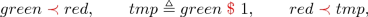where green and red are clocks respectively representing whether the green (resp. red) light is turned on, the clock tmp is an auxiliary clock used to help specify the constraints on clocks.

There exists exactly one schedule satisfying the three constraints, as shown in Fig. 2. In this schedule, the clock $$tmp$$ has the same behavior as $$green$$ from step 2, while the clock $$red$$ has the opposite behavior to $$green$$. Namely, $$red$$ and $$green$$ operates in an alternative manner. For simplicity, we also writeto denote the alternation relation of the two clocks.

Although one may be able to find one or more schedules for some simple constraints, to our knowledge, there is no generally applicable decision procedure solving the scheduling problem of full CCSL. There are two main challenges. First, schedules are essentially infinite, i.e., defined on all the natural numbers. Second, the precedence is stateful, i.e., it depends on the history, and there is no upper bound on how far in the history one must go back. It may then require an infinite memory to store the history. As a first step to tackle this challenging problem, in this work, we first consider the bounded scheduling problem.

### 3.2 Bounded Scheduling Problem

Given a bound $$k\in \mathbb {N}^+$$, let $$\sigma :\mathbb {N}^+_{\le k}\rightarrow 2^C$$ be a function. $$\sigma$$ is an k-bounded schedule of a set $$\varPhi$$ of CCSL constraints, denoted by $$\sigma \models _k\varPhi$$, iff there exists a schedule $$\delta$$ such that $$\delta (i)=\sigma (i)$$ for every $$i\in \mathbb {N}^+_{\le k}$$ and $$\delta \models \varPhi$$ from step 1 up to k, where $$\mathbb {N}^+_{\le k}:=\{1,\cdots ,k\}$$.

### Definition 6

(Bounded scheduling problem). The bounded scheduling problem is to determine, for a given set $$\varPhi$$ of CCSL constraints and a bound k, whether there is an k-bounded schedule $$\sigma$$ for $$\varPhi$$, i.e., $$\sigma \models _k\varPhi$$.

### Theorem 1

(Sufficient condition of unschedulability). If a set $$\varPhi$$ of constraints has no k-bounded schedule for some $$k\in \mathbb {N}^+$$, then $$\varPhi$$ is unschedulable.

The proof is straightforward by contradiction.

It is easy to see that the bounded scheduling problem is decidable, as there are finitely many potential k-bounded schedules, i.e., $$(2^{|C|}-1)^k$$, where |C| denotes the number of clocks. Furthermore, the satisfiability problem of Boolean formulas can be reduced to the bounded scheduling problem in polynomial time.

### Theorem 2

The k-bounded scheduling problem of CCSL is $$\mathsf{NP}$$-complete, even if $$k=1$$.

### Proof

The NP upper bound can be proved easily based on the facts that the number of possible k-bounded schedules is finite and the universal quantification $$\forall n\in \mathbb {N}^+_{\le k}$$ can be eliminated by enumerating all the possible values in $$\mathbb {N}^+_{\le k}$$.

We prove the NP-hardness by a reduction from the satisfiability problem of Boolean formulas which is known $$\mathsf{NP}$$-complete. Consider the Boolean formula $$\phi =\bigwedge _{i=1}^m(l_i^1\vee l_i^2\vee l_i^3)$$, where $$m\in \mathbb {N}^+$$ and $$l_i^j$$ for $$j\in \{1,2,3\}$$ is either a Boolean variable x or its negation $$\lnot x$$. Let $$\mathsf{Var}(\phi )$$ denote the set of Boolean variables appearing in $$\phi$$. We construct a set of CCSL constraints $$\varPhi$$ as follows.

For each $$x\in \mathsf{Var}(\phi )$$, we have two clocks $$x^+$$ and $$x^-$$. Let $$\mathsf{enc}(x)=x^+$$ and $$\mathsf{enc}(\lnot x)=x^-$$. Each clause $$l_i^1\vee l_i^2\vee l_i^3$$ in $$\phi$$ is encoded as the CCSL constraint $$c_i\triangleq \mathsf{enc}(l_i^1) + \mathsf{enc}(l_i^2)+ \mathsf{enc}(l_i^3)$$, denoted by $$\psi _i$$. Note that $$c_i\triangleq \mathsf{enc}(l_i^1) + \mathsf{enc}(l_i^2)+ \mathsf{enc}(l_i^3)$$ can be transformed into CCSL constraints by introducing one auxiliary clock c, i.e., $$\{c_i\triangleq \mathsf{enc}(l_i^1) + \mathsf{enc}(l_i^2)+ \mathsf{enc}(l_i^3)\}\equiv \{c_i\triangleq \mathsf{enc}(l_i^1) +c,c \triangleq \mathsf{enc}(l_i^2)+ \mathsf{enc}(l_i^3)\}$$.

Let $$\mathsf{enc}(\phi )$$ denote the following set of CCSL constraints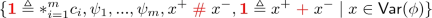where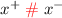and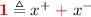enforce that either $$x^+$$ or $$x^-$$ ticks at each step, but not both. This encodes that either x is true or $$\lnot x$$ is true. Note that $$\tau \triangleq *_{i=1}^m c_i$$ is a shorthand of $$\tau \triangleq c_1*\cdots *c_m$$, and can also be expressed in CCSL constraints by introducing polynomial number of auxiliary clocks. For instance, $$\{c \triangleq c_1*c_2*c_3\}\equiv \{c \triangleq c_1*c',c'\triangleq c_2*c_3\}$$. We can show that $$\phi$$ is satisfiable iff $$\mathsf{enc}(\phi )$$ is 1-bounded schedulable. The satisfiability problem of Boolean formulas is NP-complete, we get that the 1-bounded scheduling problem of CCSL is NP-hard. The k-bounded scheduling problem for $$k>1$$ immediately follows by repeating the ticks of clocks at the first step.    $$\square$$

Theorem 2 indicates the time complexity of the bounded scheduling problem. Thus, we need to find practical solutions that are algorithmically efficient for it. In the next section, we propose an SMT-based decision procedure for the bounded scheduling problem and a sound algorithm for the scheduling problem. Thanks to advances in state-of-the-art SMT solvers such as Z3 , our approach is usually efficient in practice.

## 4 Decision Procedure for the Scheduling Problem

### 4.1 Transformation from CCSL into SMT

Let us fix a set of CCSL constraints $$\varPhi$$ defined over a set C of clocks. Each clock $$c\in C$$ is interpreted as a predicate $$t_c:\mathbb {N}^+\rightarrow \mathsf{Bool}$$ such that for all $$i \in \mathbb {N}^+$$, $$t_c(i)$$ is true iff the clock c ticks at i, where $$\mathsf{Bool}$$ denotes Boolean sort. A schedule $$\delta$$ of $$\varPhi$$ is encoded as a set of predicates $$\mathcal {T}_C=\{t_c|c\in C\}$$ such that the following condition holds: for all $$t_c\in \mathcal{T}_C$$,

\begin{aligned} \forall i\in \mathbb {N}^+. t_c(i)\Leftrightarrow c\in \delta (i). \end{aligned}

Recalling that schedules forbid stuttering steps, this condition is enforced by restricting the predicates $$t_{c}$$ in $$\mathcal {T}_C$$ to satisfy the following condition: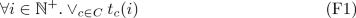Formula F1 specifies that at each step i at least one clock c ticks, i.e., $$t_c(i)$$ holds.

For each clock $$c\in C$$, we introduce an auxiliary function $$h_c:\mathbb {N}^+\rightarrow \mathbb {N}$$ to encode its history. For each $$i\in \mathbb {N}^+$$,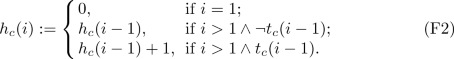Intuitively, $$h_c(i)$$ is equivalent to $$\chi (c,i)$$ for each $$i\in \mathbb {N}^+$$. The set of all the auxiliary functions is denoted by $$\mathcal {H}_C$$.

By replacing each occurrence of clock c in $$\delta (n)$$ (resp. $$c\not \in \delta (n)$$) with $$t_c(n)$$ (resp. $$\lnot t_c(n)$$) and $$\chi (c,n)$$ with $$h_c(n)$$ in the definition of each CCSL constraint, each CCSL constraint $$\phi$$ can be encoded as an SMT formula $$\llbracket \phi \rrbracket$$.

We use $$\llbracket \varPhi \rrbracket$$ to denote the conjunction of Formulas F1F2 and the SMT encodings of CCSL constraints in $$\varPhi$$. Formally,

\begin{aligned} \llbracket \varPhi \rrbracket :=\text {F1}\wedge \text {F2}\wedge (\wedge _{\phi \in \varPhi }\llbracket \phi \rrbracket ). \end{aligned}

Finding a schedule for $$\varPhi$$ amounts to finding a solution, i.e., definitions of predicates in $$\mathcal {T}_C$$, which satisfies $$\llbracket \varPhi \rrbracket$$.

### Proposition 1

$$\varPhi$$ has a schedule iff $$\llbracket \varPhi \rrbracket$$ is satisfiable.

The scheduling problem of $$\varPhi$$ is transformed into the satisfiability problem of the formula $$\llbracket \varPhi \rrbracket$$. However, according to the SMT-LIB standard , $$\llbracket \varPhi \rrbracket$$ belongs to the logic of UFLIA (formulas with Uninterpreted Functions and Linear Integer Arithmetic), whose satisfiability problem is undecidable in general. Nevertheless, the SMT encoding is still useful to solve the bounded scheduling problem, which we will present in the next subsection.

### 4.2 Decision Procedure for the Bounded Scheduling Problem

For k-bounded scheduling problem, it suffices to consider schedules $$\delta :\mathbb {N}^+_{\le k}\rightarrow 2^C$$. Moreover, the quantifiers in $$\llbracket \varPhi \rrbracket$$ can be eliminated once the bound k is fixed. Hence, we can resort to state-of-the-art SMT solvers. Formally, let $$\llbracket \varPhi \rrbracket _k$$ be the formula obtained from $$\llbracket \varPhi \rrbracket = F1 \wedge F2 \wedge (\bigwedge _{\phi \in \varPhi }\llbracket \phi \rrbracket )$$ by

• restricting the domain of predicates $$t_c\in \mathcal {T}_C$$ and functions $$h_c\in \mathcal {H}_C$$ to $$\mathbb {N}^+_{\le k}$$;

• replacing quantifications $$\forall n\in \mathbb {N}^+$$ and $$\exists m\in \mathbb {N}^+$$ with $$\forall n\in \mathbb {N}^+_{\le k}$$ and $$\exists m\in \mathbb {N}^+_{\le k}$$ in $$(\bigwedge _{\phi \in \varPhi }\llbracket \phi \rrbracket )$$.

### Proposition 2

$$\varPhi$$ is k-bounded schedulable iff $$\llbracket \varPhi \rrbracket _k$$ is satisfiable.

Moreover, if $$\llbracket \varPhi \rrbracket _k$$ is satisfiable, then $$\llbracket \varPhi \rrbracket _{k'}$$ is satisfiable for all $$k'\le k$$.

### 4.3 A Sound Algorithm for the Scheduling Problem

According to Theorem 1, Propositions 1 and 2, (1) if $$\llbracket \varPhi \rrbracket$$ is satisfiable, then $$\varPhi$$ is schedulable, and (2) if $$\llbracket \varPhi \rrbracket _k$$ for some $$k \in \mathbb {N}^+$$ is unsatisfiable, then $$\varPhi$$ is unschedulable. We can deduce a sound algorithm for checking the general scheduling problem. However, randomly choosing a bound k and checking whether or not $$\llbracket \varPhi \rrbracket _k$$ is unsatisfiable may be inefficient, as the k-bounded scheduling problem is NP-hard (cf. Theorem 2), and larger bound k may result in time out, but smaller bound k may result in that $$\llbracket \varPhi \rrbracket _k$$ is satisfiable. Indeed, if we consider the maximal bound B, then the random approach may have to call SMT solving $$\mathbf{O}(B)$$ times. Alternatively, we propose a binary-search based approach as shown in Algorithm 1 for a given maximal bound B, which invokes SMT solving at most $$\mathbf{O}(|\log _2B|)$$ times.

Given a set $$\varPhi$$ of constraints in CCSL, a timeout threshold T and a maximal bound B, Algorithm 1 first invokes an SMTSolver to decide whether $$\llbracket \varPhi \rrbracket$$ is satisfiable or not within $$\mathsf T$$ time. If $$\llbracket \varPhi \rrbracket$$ is satisfiable, then Algorithm 1 returns (SAT,0), meaning that $$\varPhi$$ is schedulable. Otherwise, it binary searches a bound $$k\le B$$ such that $$\llbracket \varPhi \rrbracket _{k}$$ is satisfiable while $$\llbracket \varPhi \rrbracket _{k+1}$$ (if $$k+1\le B$$) is unsatisfiable or cannot be verified in time T.

### Theorem 3

Algorithm 1 has the following three properties:

1. 1.

If it returns $$(\mathtt{SAT,0})$$, then $$\varPhi$$ is schedulable.

2. 2.

If it returns $$(\mathtt{UNSAT, k})$$, then $$\varPhi$$ is unschedulable. If $$k\ne 0$$, then $$\varPhi$$ has k-bounded schedulable, otherwise does not have any bounded schedulable.

3. 3.

If it returns $$(\mathtt{Timeout, k})$$, then $$\varPhi$$ is k-bounded schedulable if $$k\ne 0$$, otherwise no bounded schedule is found for $$\varPhi$$.

## 5 Case Study and Performance Evaluation

We implemented our approach in a prototype tool with Z3  as its underlying SMT solver. We conduct a case study on expressing requirements of an interlocking system in CCSL constraints and analyzing its schedulability. Then, we prove 12 algebraic properties of CCSL constraints using the tool. Finally, we evaluate the performance of the tool using 9 sets of CCSL constraints.

### 5.1 Schedulability of an Interlocking System

The interlocking system is a subsystem of a rail transit system. It is used to prevent trains from collisions and derailments when they are moving under the control of signal lights. As shown in Fig. 3, the interlocking system monitors the occupancy status of the individual track section, and sends signals to inform drivers whether they are allowed to enter the route or not. The railway tracks are divided into sections. Each section is associated with a track circuit for detecting whether it is occupied by a train or not. Signal lights are placed between track sections. They can be red and green to indicate proceeding and stopping, respectively.

The mechanism and operation procedure of the interlocking system are summarized as follows.

1. 1.

To enter a track, a train first sends a request to the control center.

2. 2.

On receiving the request, the control center sends an inquiry to the track circuit to detect the status of the track.

3. 3.

If the track is occupied, it sends checkFail to the control center, and otherwise checkSucc.

4. 4.

On receiving the message checkFail (resp. checkSucc), the control center sends a red (resp. green) signal pulse to the signal light.

5. 5.

The signal light turns red (resp. green) on receiving the red (resp. green) signal pulse.

6. 6.

The train will enter after seeing the light is green, and the track becomes occupied. In case of the red light, the train must stop and wait.

7. 7.

The track becomes unoccupied after the train leaves. If the train is waiting, it must send a request again after some time.

There are time constraints on the above operations. For instance, the control center needs to get a response from the track circuit within 30 ms after sending an inquiry to it. The train must make decision within 50 ms after it sends a request to the control center. The light should turn to the corresponding color within 30 ms after it receives a pulse. After the track becomes occupied (resp. unoccupied), the light must turn red (resp. green) within 40 ms.

Table 2 shows the main logical constraints on the operations in the system and their timing constraints. We use some non-standard constraint expressions for the sake of compactness. Constraint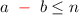denotes that b must tick within n steps after a ticks. It equals the set of the following three constraints: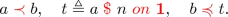Note that in this example the unit of time is millisecond (ms). Thus, there is an implicit assumption in the constraints that every tick of a logic clock means the elapse of one millisecond.

Most constraints in Table 2 are straightforward, except the six constraints marked with waved underlines. The first three constraints specify that $$\mathtt {checkFail}$$ only can occur between the occurrences of $$\mathtt {getUnoccupied}$$ and $$\mathtt {getOccupied}$$. The others specify the following two requirements:

1. 1.

$$\mathtt {checkSucc}$$ only can occur after $$\mathtt {getUnoccupied}$$ and before $$\mathtt {getOccupied}$$;

2. 2.

$$\mathtt {getUnoccupied}$$ precedes $$\mathtt {getOccupied}$$.

Given these constraints, our tool found a bounded schedule as depicted in Fig. 4. From step 1 to step 7, one complete process is finished. Initially, the track gets unoccupied. At step 2, a request is made, which causes subsequent operations to occur from step 3 to step 7. At step 29, a fail case occurs because another train enters (step 26) but has not left (step 31). The train that made the request has to wait (step 33).

If we extend the bounded schedule by infinitely repeating the behaviors of all the clocks between step 51 and 69 from step 70, we obtain an infinite schedule. The extended schedule satisfies all the constraints, and thus it is a witness of the schedulability of designed mechanism for the interlocking system.

In this paper, we are only concerned with the schedulability of the constraints in the example. Some other kinds of temporal properties also need to verify. For instance, we must guarantee that whenever a train requests to enter the station, it must eventually enter. We also need to verify the system is deadlock-free. Such temporal properties can be verified by LTL model checking of CCSL constraints using SMT technique . We omit it because it is beyond the scope of this paper.

### 5.2 Automatic Proof of CCSL Algebraic Properties

Using the proposed approach, we can also prove automatically algebraic properties of CCSL constraints such as the commutativity of exclusion and transitivity of causality. Algebraic properties of CCSL constraints can be represented as $$\varPhi \Rightarrow \phi$$, where $$\varPhi$$ is a set of CCSL constraints and $$\phi$$ is a constraint derived from $$\varPhi$$. Proving $$\varPhi \Rightarrow \phi$$ is valid equals proving the unsatisfiability of $$\llbracket \varPhi \rrbracket \wedge \lnot \llbracket \phi \rrbracket$$, which can be solved by Algorithm 1.

Let us consider the proof of the slowestness of infimum as an example. The slowestness of infimum means that an infimum constraint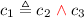defines the slowest clock $$c_1$$ among those that are faster than both $$c_2$$ and $$c_3$$.

### Proposition 3

(Slowestness of infimum). Given two clocks $$c_2,c_3$$, letand $$c_4$$ be an arbitrary clock such that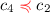and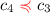, then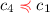.

This is proved by transforming CCSL constraints into the following SMT formula according the SMT encoding method: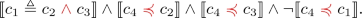Algorithm 1 returns (UNSAT, 0), which means that the formula is proved unsatisfiable. The proposition is proved.

Table 3 lists the algebraic properties that have been successfully proved in our approach. Algebraic properties are useful to help understand the relation among CCSL constraints. Using them we can also verify whether some CCSL constraints are redundant or inconsistent for a given set of CCSL constraints.

### 5.3 Performance Evaluation

To evaluate the performance our tool, we collected 9 sets of CCSL constraints from the literature and real-world applications, and analyzed their schedulability using our tool. Under different time thresholds, we calculate the maximal bounds under which the constraints are schedulable.

Table 4 shows all the experimental results including the corresponding execution time. All the experiments were conducted on a Win 10 running on an i7 CPU with 2.70 GHz and 16 GB memory. The numbers followed by asterisks are the maximal bounds such that the corresponding constraints are bounded schedulable, but unschedulable in the next step. It is interesting to observe from Table 4 that time cost is loosely related to size (the number of clocks and constraints), thanks to efficient search strategies of SMT solvers. This is in striking contrasts to automata-based [29, 35] and the rewriting-based approaches , whose scalability suffers from both the numbers of clocks and constraints.

## 6 Related Work

CCSL is directly derived from the family of synchronous languages, such as Lustre , Esterel  and Signal , and its the scheduling problem of CCSL is akin to what synchronous languages call clock calculus. The main differences are: CCSL is a specification language, while others are programming languages; and CCSL partially describes what is expected to happen in a declarative way and does not give a direct operational deterministic description of what must happen. Furthermore, CCSL only deals with pure clocks while the others deal with signals and extract the clocks when needed.

The Esterel compiler  applies a constructive approach to decide when a signal must occur (compute its clock) and what its value should be. This requires a detection of causality cycles, or intra-cycle data dependencies, which are also naturally addressed by our approach. However, the Esterel compiler compiles an imperative program into a Boolean circuit, or equivalently a finite state machine. Consequently, it cannot deal with CCSL unbounded schedules.

The clock calculus in Signal attempts to detect whether the specification is endochronous , in which case it can generate some efficient code. This analysis is mainly based on the subclock relationship that also exists in CCSL. In CCSL, we consider the problem whether there is at least one possible schedule or not.

In Lustre and its extensions, clocks are regarded as abstract types  and the clock calculus computes the relative rates of clocks while rejecting the program when computing the rates is not possible. In most cases, the compiler attempts to build bounded buffers and to ensure that the functional determinism can be preserved with a finite memory. In our case, we do not seek to reach a finite representation, as in the first specification steps this is not a primary goal for the designers. Indeed, this might lead to an over-specification of the problem.

Classical real-time scheduling problem  usually relies on task models, arrival patterns and constraints (e.g., precedence, resources) to propose algorithms for the scheduling problem with analytical results  or heuristics depending on the specific model (e.g., priorities, preemptive). Other solutions, based on timed automata [1, 2, 17] or timed Petri nets [8, 18], propose a general framework for describing all the relevant aspects without assuming a specific task model. CCSL offers an alternative method based on logical time. It is believed that logical time and multiform time bases offer some flexibility to unify functional requirements and performance constraints. We rely on CCSL and we claim that after encoding a task model in CCSL, finding a schedule for the CCSL model also gives a schedule for the encoded task model .

There have been many efforts made towards the scheduling problem of CCSL, though no conclusion is drawn on its decidability. TimeSquare  is a simulation tool for CCSL which can produce a possible schedule for a given set of CCSL, up to a given user-defined bound. It also supports different simulation strategies for producing desired execution traces. Some earlier work  define the notion of safe CCSL specifications that can be encoded with a finite-state machine. The scheduling problem is decidable for safe specifications, as one can merely enumerate all the (finite) solutions. A semi-algorithm can build the finite representation when the specification is safe . In , Zhang et al. proposed a state-based approach and a sufficient condition to decide whether safe and unsafe specifications accept a so-called periodic schedule . This allows to build a finite solution for unsafe specifications, while there may also exist infinite solutions. Xu et al. proposed a notion of divergence of CCSL to study the schedulability of CCSL, and proved that a set of CCSL constraints is schedulable if all the constraints are divergent . They resorted to the theorem prover PVS  to assist the divergence proof.

The scheduling problem of CCSL constraints in this work resorts to SMT solving to deal with the bounded and unbounded schedules. Using SMT solving has two advantages: (1) it is usually efficient in practice, and (2) it can deal with unsafe CCSL constraints such as infimum and supremum .

Some basic algebraic properties on CCSL relations have been established manually before  but we provide here an automatic framework to do so.

## 7 Conclusion and Future Work

In this work, we proved that the bounded scheduling problem of CCSL is NP-complete, and proposed an SMT-based decision procedure for the bounded scheduling problem. The procedure is sound and complete. The experimental results also show its efficiency in practice. Based on this decision procedure, we devised a sound algorithm for the general scheduling problem. We evaluated the effectiveness of the proposed approach on an interlocking system. We also showed our approach can be used to prove algebraic properties of CCSL constraints.

Our approach to the bounded scheduling problem of CCSL makes us one step closer to tackling the general (i.e. unbounded) scheduling problem. As the case study demonstrates, one may find an infinite schedule by extending a bounded one such that the extended infinite schedule still satisfies the constraints. This observation inspires future work to investigate mechanisms of finding such bounded schedules, hopefully with SMT solvers by extending our algorithm. In our earlier work , we proposed a similar approach to search for periodical schedules in bounded steps. In that approach, CCSL constraints are transformed into finite state machine and consequently suffers from the state explosion problem. We believe our SMT-based approach can be extended to their work while still avoiding state explosion. We leave it to future work.